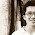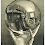## Wednesday, September 09, 2009

### 09 09 09 Twins

Suppose that 3% of all births give rise to twins.  If 1,000 people are born on 09/09/09 in the state of New York, how many do you expect will be twins?

BTW, be careful today searching out numerology trivia.  I did and immediately landed on a malware site.

1.At first I thought, "Wow, this is insanely easy." But then I read it again, and I THINK the answer is 60 twins (thus, 30 births which each yielded twins).

2.60 twins.
3% of 1000 = 30 births x 2= 60
Hope i am right... ;-)

3.But is is said that 1000 PEOPLE are brn. so my guess is 30... :-/

4.I think it's 58.

I'm about to do some incorrect math, but it's the logic of the matter that I want to highlight here.

Assumong 30 sets of twins, like we did, that means there were actually only 970 BIRTHS to yeild 1000 babies.

so .03x970=29.1 sets of twins. It's an approximation since we used incorrect (albeit easy) math to get the original 970, but since we can't have .1 sets of twins, we round down, and then doubling we get 58 twins.

Of course, I could be overthinking it.

5.I agree with Jolson. Let me expand the math:

Let X represent the number of non-twin-yielding births on 9/9/09, and let Y represent the number of twin-yielding births. It's pretty clear that the total number of people born on this day is X + 2Y (assuming no triplets, etc). We're told that this quantity is equal to 1,000. So we have:
1,000 = X + 2Y

Now, we're also told that "3% of all births give rise to twins". With X and Y defined as above, this means that we expect the value of Y (the number of twin-yielding births) to equal 3% of the total number of births (X+Y). Mathematically, that is (and proceeds to be):
Y = 0.03 * (X + Y)
Y = 0.03X + 0.03Y
0.97Y = 0.03X
Y = (0.03/0.97)X

This ratio arises strictly from the proportions of births given.

Substituting into our first equation, we have, progressively:
1,000 = X + 2Y
1,000 = X + 2(0.03/0.97)X
1,000 = (0.97/0.97)X + (0.06/0.97)X
1,000 = (1.03/0.97)X
X = 1000 * (0.97/1.03) = 941.75...

Now, 2Y = 1,000 - X = 58.25,
so Y = 29.125.

We expect 942 no-twin births, and 29 twin-births, for a total of 942 + 29*2 = 1,000 people born. 29*2 = 58 of those people are twins.

Quick verification: 29/(942+29) = .02986, the closest to 3% we can come using whole people...

6.Ya I agree with Jolson.
There are 59 twins.
www.guessthelogo.blogspot.com

7.Oudeis, that was a great explanation and one I can't top. We should expect 58 twins, that is, probability-wise (is that an expression?).

Leave your answer or, if you want to post a question of your own, send me an e-mail. Look in the about section to find my e-mail address. If it's new, I'll post it soon.

Please don't leave spam or 'Awesome blog, come visit mine' messages. I'll delete them soon after.

Enter your Email and join hundreds of others who get their Question of the Day sent right to their mailbox

The Lamplight Manor Puzz 3-D